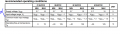# Had a trouble understanding the input voltage range of datasheet of OPAMP TLV2442AQPWR.

#### ashokraj

Joined Feb 1, 2018
135
I would like to know the following:
1. what is the input voltage range of power supply i could give to this opamp.
2. lets say if i give -3.3V what is the VDD+ i will have to give ?
I have a trouble understanding this. Below is from the datasheet of TLV2442aqpwr.
For example if i consider Q suffix, does it mean if i give -2.7 to negative power supply of opamp , max i can give to positive power supply of opamp is =2.7-1.3 --->=1.4!!!?#### Attachments

• 1.8 MB Views: 4

#### Papabravo

Joined Feb 24, 2006
20,623
1. The power supply range is given as ± 12 VDC. So for example if I use +4.5 VDC and -0.8 VDC as my supply rails the input range would be [-0.8 VDC, 3.5 VDC] for the C suffix part
2. If Vi = -3.3 V then Vdd- must be -3.3 VDC or less. Vdd+ can be anything between say 2.7 VDC and +12 VDC
No, the positive supply must be in the range [2.7, 10] as a recommended operating condition. The negative supply is not similarly restricted. Whatever it is, that will be the minimum input voltage. Also the input mist be some amount , depending on the suffix below the positive rail, whatever that is.

Last edited:

#### Audioguru again

Joined Oct 21, 2019
6,461
The OP asked, "What is the input voltage range of the power supply?". The datasheet recommends a total power supply of 10V max. A negative supply is not needed, it works fine with 0V and +2.7V to +10V.
He is confusing the power supply voltage range with the input voltage range.

For the Q version, if the negative power supply is -2.7V then the max positive supply is 10V - 2.7V= +7.3V.
The recommended input voltage range is +2V above the negative supply voltage to -1.3V below the positive supply voltage.

•ashokraj and OBW0549

#### ashokraj

Joined Feb 1, 2018
135
The OP asked, "What is the input voltage range of the power supply?". The datasheet recommends a total power supply of 10V max. A negative supply is not needed, it works fine with 0V and +2.7V to +10V.
He is confusing the power supply voltage range with the input voltage range.

For the Q version, if the negative power supply is -2.7V then the max positive supply is 10V - 2.7V= +7.3V.
The recommended input voltage range is +2V above the negative supply voltage to -1.3V below the positive supply voltage.
For a power supply voltage is it recommended to give -3.3v and +4.7V??

#### Audioguru again

Joined Oct 21, 2019
6,461
I do not see why they recommend that the input should not go within 2V from the negative supply voltage.

#### MisterBill2

Joined Jan 23, 2018
16,635
Certainly there must be a lot more to the data sheet, such as typical operating conditions. The rest of the data sheet is where to find the answer.